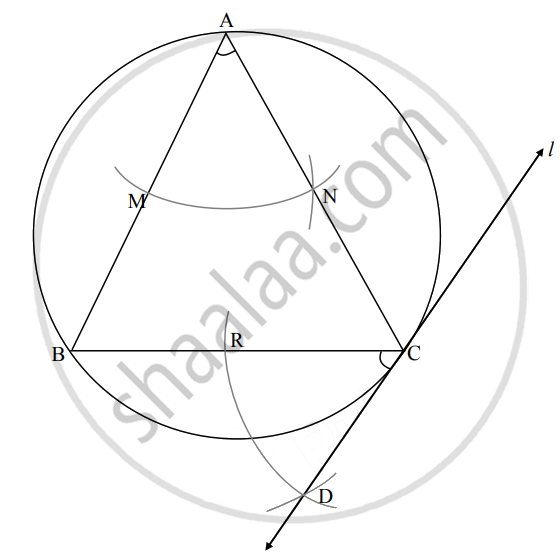# Do the following activity to draw tangents to the circle without using center of the circle. a. Draw a circle with radius 3.5 cm and take any point C on it.b. Draw chord CB and an inscribed angle CAB - Geometry

Diagram

Do the following activity to draw tangents to the circle without using the center of the circle.

1. Draw a circle with radius 3.5 cm and take any point C on it.
2. Draw chord CB and an inscribed angle CAB.
3. With the center A and any convenient radius, draw an arc intersecting the sides of angle BAC in points M and N.
4. Using the same radius, draw an arc intersecting the chord CB at point R.
5. Taking the radius equal to d(MN) and center R, draw an arc intersecting the arc drawn in the previous step. Let D be the point of intersection of these arcs. Draw line CD. Line CD is the required tangent to the circle.

#### SolutionConcept: Construction of a Tangent to the Circle at a Point on the Circle
Is there an error in this question or solution?

Share